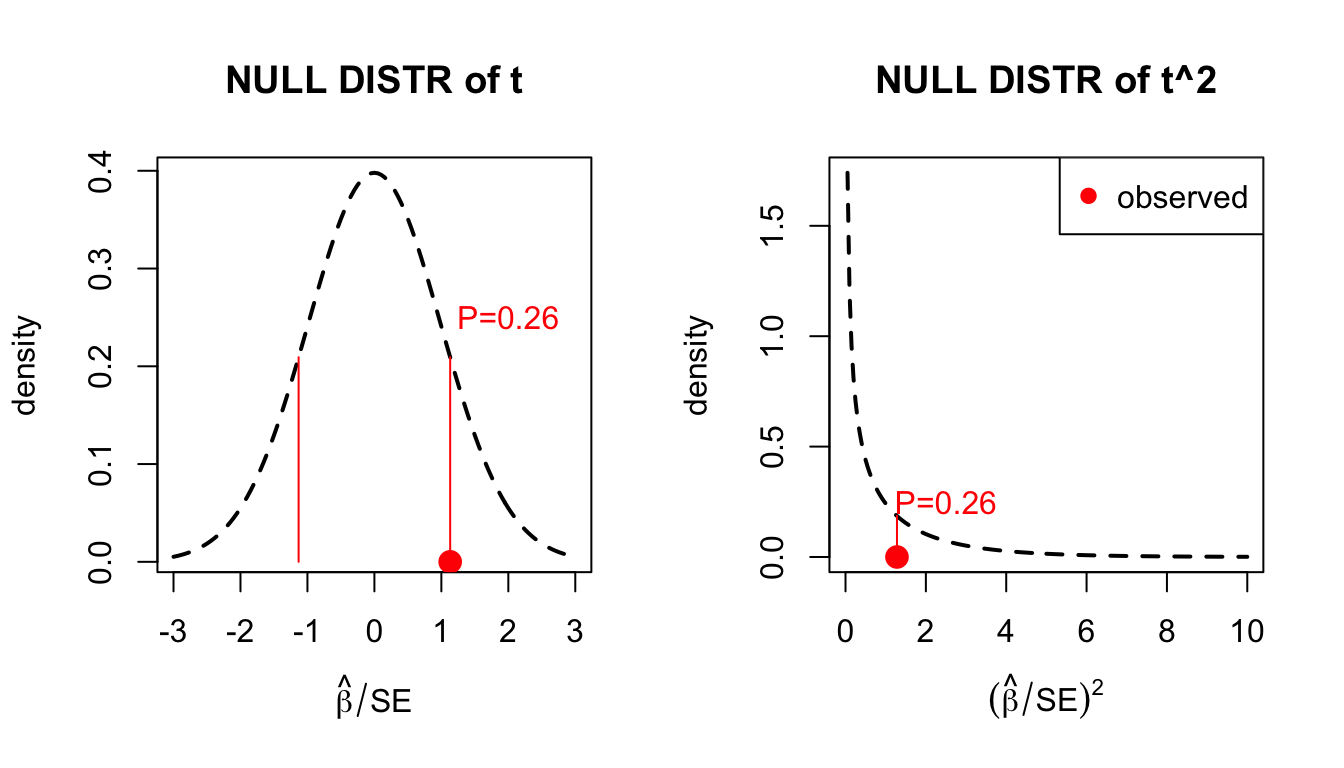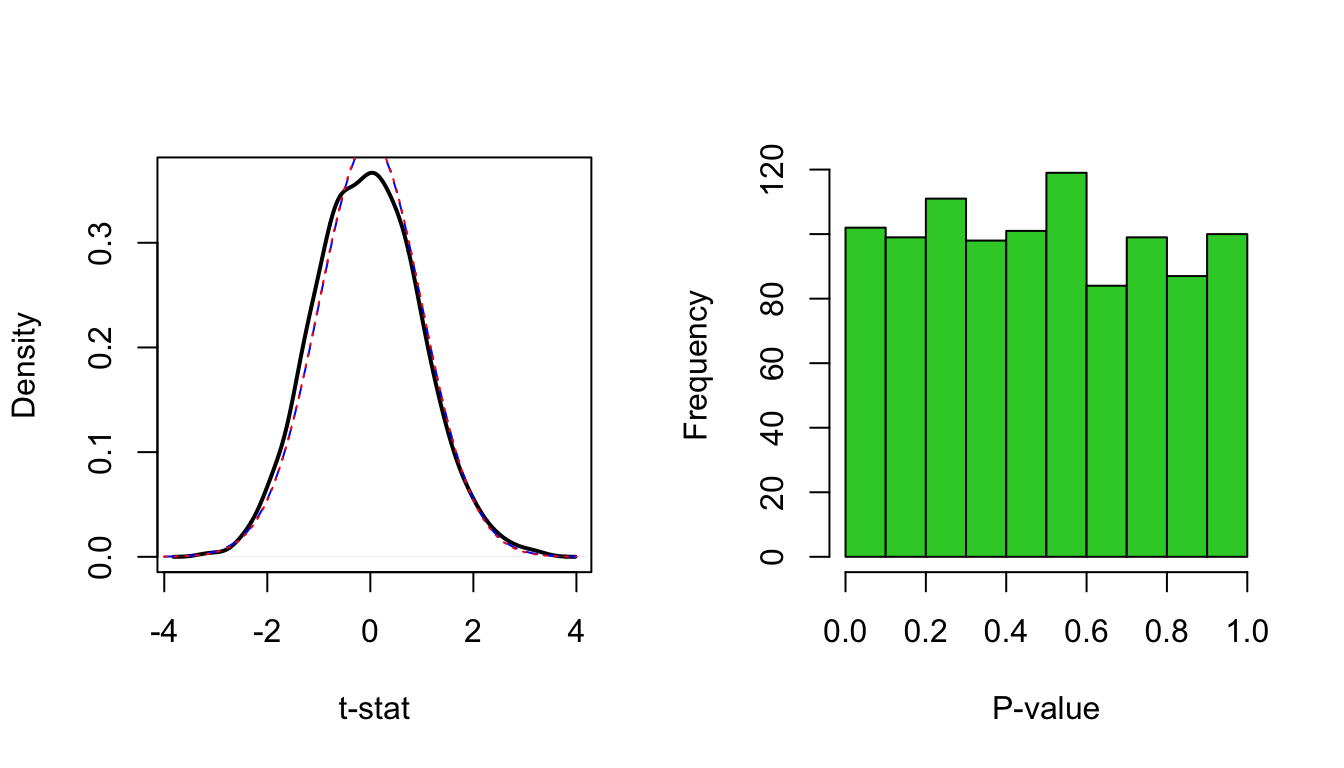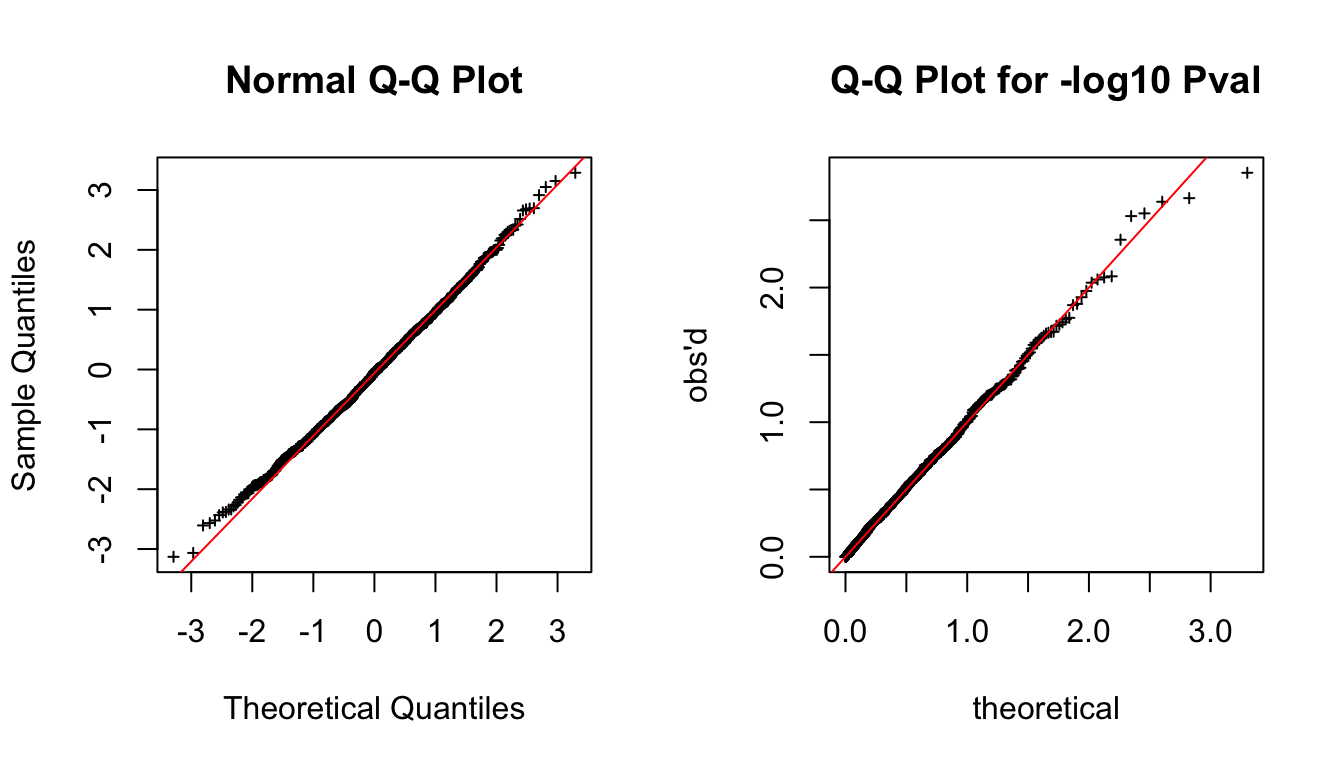The slide set referred to in this document is “GWAS 2”.

A GWAS conducted by the additive regression model returns three quantities for each variant:

• $$\widehat{\beta}$$, the effect size estimate for allele 1, also called effect allele;
• SE of $$\widehat{\beta}$$, describing uncertainty in $$\widehat{\beta}$$;
• P-value.

We saw earlier how to interpret $$\beta$$ as a difference between the means of the adjacent genotype groups, and how SE describes the uncertainty of the estimate $$\widehat{\beta}$$ (slide 2). These two parameters tie the data to the actual phenotypic change in a concrete way. For example, from these quantities we could infer that a variant increases LDL levels by 0.5 mmol/L (95%CI 0.42..0.58) or that another variant increases odds of MS-disease by 20% (95%CI 16%…24%). While these are the most concrete and detailed information about the effect of a variant available in GWAS output, still, typically, the first statistic we look at is the P-value.

P-value has a central role in GWAS because the null hypothesis of exactly zero effects is (thought to be) a realistic hypothesis for a large majority of all variants tested, and a crude assessment of zero vs. non-zero effect can be done based on the P-value. Additionally, a genotype-phenotype association is interesting primarily because it points to biology behind the phenotype whereas its effect size may be considered secondary to the existence of an association. This is also true because typically individual GWAS findings have effect sizes that are so small that they alone do not have any direct clinical use. Therefore, the magnitude of the effect size has a smaller role in statistical inference in the GWAS context than in many other contexts, such as, e.g., in social sciences, where it is unrealistic to expect that any effect is exactly zero. In those other research fields, the focus should be on the size of the effect whereas there P-value is only a secondary quantity.

2.1 What is a P-value? (slides 3-6 & 18-21)

The purpose for using P-value is to evaluate whether the observed data seem inconsistent with the null hypothesis. Typically, the null hypothesis states that the variant is not important, or technically, that its effect size is 0. We have one null hypothesis per each variant. P-value is a probability of getting something “at least as extreme” as what has been observed, if the null hypothesis was true. Therefore, small P-value is taken as evidence that the null hypothesis may not be true. Logic goes that if P-value is very small, then it would be very unlikely to observe the data at hand under the null hypothesis – and therefore either null hypothesis is not true or we have encountered an unlikely event. P-value is thus a simple numerical summary of the consistency between the null hypothesis and the observed data. But note that P-value is NOT a probability that the null hypothesis is true: P-value is probability of data given a hypothesis not a probability of the hypothesis given data (slides 8-9).

Let’s do one linear regression and put its P-value in its place in the null distribution of t-statistic. The goal is to study whether the effect $$\beta$$ of the additive GWAS model $$y=\mu + x \beta + \varepsilon$$ is zero. The null hypothesis is $$H_0: \beta =0$$. Here P-value tells that if the true slope $$\beta=0$$, what is the probability that we observe a data set from which the computed slope is at least as large (in absolute value) as the observed estimate $$\widehat{\beta}$$. Most often we don’t look at the null distribution of $$\widehat{\beta}$$, which depends on sample size and variances of $$x$$ and $$\varepsilon$$, but instead we look at the null distribution of the t-statistics $$t=\widehat{\beta}/\textrm{SE}$$ which has distribution $$t(n-2)$$, i.e., t-distribution with $$n-2$$ degrees of freedom, where $$n$$ is the sample size. When $$n>50$$, we can approximate $$t(n-2)$$ with the standard normal distribution $$\mathcal{N}(0,1)$$, and this approximation is typically done in GWAS setting where t-statistic is often called z-score that refers to standard normal variable. The other way to express the same normal approximation is to say that the square of the t-statistic follows a chi-square distribution with 1 degree of freedom: $$t^2 \sim \chi_1^2$$. By squaring $$t$$, this approach ignores the sign of the estimate, which is not a problem in the GWAS setting, since we do not by default have any prior knowledge on the direction of the effect. Let’s draw pictures about P-values using both $$t$$ and $$t^2$$ statistics.

n = 100
f = 0.3 #MAF
x = rbinom(n, 2, f) #example genotypes for n individuals
y = rnorm(n) #outcome that is independent of x
lm.fit = lm( y ~ x )
par( mfrow = c(1,2) ) #draw 2 panels on the grid with 1 row and 2 cols
#1st on t-statistic's scale
x.grid = seq(-3, 3, 0.05) #we need this to define the plotting region
plot(x.grid, dt(x.grid, df = n-2), lty = 2, lwd = 2, t = "l",
xlab = expression( hat(beta)/SE ), ylab = "density", main="NULL DISTR of t") #null distr. of t-stat.
t.stat = summary(lm.fit)$coeff[2,3] #t-statistic: Estimate/SE points(t.stat, 0, pch = 19, cex = 1.5, col = "red") segments(t.stat*c(1,-1), c(0,0), t.stat*c(1,-1), rep( dt( t.stat, df = n-2), 2 ), col = "red") text(2, 0.25, paste0("P=",signif(summary(lm.fit)$coeff[2,4],3)), col = "red")

#2nd on t^2 statitstic's scale
x.grid = seq(0, 10, 0.05) #we need this to define the plotting region
plot(x.grid, dchisq( x.grid, df = 1 ), lty = 2, lwd = 2, t = "l",
xlab = expression(( hat(beta)/SE)^2 ), ylab = "density", main = "NULL DISTR of t^2") #null distribution of t^2-stat.
t2.stat = summary(lm.fit)$coeff[2,3]^2 #t^2-statistic: (Estimate/SE)^2 points(t2.stat, 0, pch = 19, cex = 1.5, col = "red") segments(t2.stat, 0, t2.stat, dchisq(t2.stat, df = 1), col = "red") text(2.5, 0.25, paste0("P=", signif(summary(lm.fit)$coeff[2,4],3)), col = "red")
legend("topright", pch = 19, col = "red", leg = "observed" )P-value is the probability mass outside the red segments, i.e., on the left panel it is the sum of the two tail probabilities and on the right panel it is the right tail probability. It tells how probable, under the null, it is to get at least as extreme (from 0) observation as we have got here. Note that chi-square distribution leads to a simpler setting since we need to consider only the right tail of the distribution to compute the P-value. For the normal distribution we need to account also for the other tail that has the different sign from the observed value of the statistic (here the left tail).

Let’s make sure that we understand where the P-value came from and let’s compute it manually from both the standard normal distribution and from the chi-square distribution using the cumulative density functions.

z = summary(lm.fit)$coeff[2,3] #t-statistic also called z-score under Normal approximation pnorm(-abs(z), 0, 1, lower = T) + pnorm(abs(z), 0, 1, lower = F) #P-value from N(0,1): left + right tail ##  0.2577372 pchisq(z^2, df = 1, lower = F) #P-value from chi-square is the upper tail ##  0.2577372 In this example, the P-value was 0.26. To interpret this value through a frequentist inference framework, we imagine an experiment where we were repeatedly to sample new data at this SNP without a real genotype-phenotype association (i.e. the true effect size was exactly zero). We would observe that in about 26% of those data sets the effect size estimate would be at least as large in absolute value as what we have observed here. We conclude that these data do not make us believe strongly in a true genotype-phenotype association, because effect size estimates at least this large in absolute value are present already in about 1/4 of the null data sets. Hence these data seem completely plausible to have originated from the null. IMPORTANT: Make sure you understand how the above interpretation of P-value is different from a wrong interpretation that based on the P-value only we could say that the probability that this SNP is null is 26% (slides 8-9). If P=0.26 is not yet a convincing association, then how small a P-value should make us reasonably convinced about an interesting genotype-phenotype association? 2.2 Distribution of P-values Let’s make data on 1000 null variants (i.e. zero effects) measured on 100 individuals and look at their P-value distributions. set.seed(39) n = 100 #individuals p = 1000 #variants measured on each individual f = 0.4 #MAF is assumed the same for all variants; doesn't actually matter here X = matrix(rbinom(n*p, 2, f), nrow = n, ncol = p) #just random genotypes y = rnorm(n) #phenotype that is not associated with any of genotypes #apply lm to each column of X separately and collect results for genotype (row 2 of coeff) lm.res = apply(X, 2 , function(x) summary(lm(y ~ x))$coeff[2,])
#result has 4 rows: beta, SE, t-stat and pval
pval = lm.res[4,] #pick pvalues

par(mfrow = c(1,2))
plot(density(lm.res[3,]), sub = "", xlab = "t-stat", main = "", lwd = 2) #should be t with n-2 df
x.seq = seq(-4,4,0.1) #x-coordinates for plotting
lines(x.seq, dt(x.seq, df = n-2), col = "blue", lty = 2) #t distribution in blue
lines(x.seq, dnorm(x.seq), col = "red", lty = 3) #normal distribution in red
hist(pval, breaks = 10, xlab = "P-value", main = "", col = "limegreen") #should be uniformly distributedpar(mfrow = c(1,2)) #Let's make qqplots for t-stats and for P-values
qqnorm(lm.res[3,], cex = 0.5, pch = 3) #t with ~100 df ~ normal, hence qqnorm()
qqline(lm.res[3,], col = "red")

#For P-values, we want to compare to the Uniform(0,1) distribution:
#We use ppoints(p) to get
#p equally spaced values in (0,1) to represent quantiles of Uniform(0,1).
#we take -log10 transformation to see the small P-values particularly well
qqplot(-log10(ppoints(p)), -log10(pval), xlab = "theoretical",
ylab = "obs'd", main = "Q-Q Plot for -log10 Pval", cex = 0.5, pch = 3)
abline(0, 1, col = "red")What are QQ-plots?

Why are P-values distributed as Uniform(0,1) under the null? $\textrm{Pr}(P \leq q_0)=\textrm{Pr(test stat falls within the most extreme region of prob. mass }q_0\, |\, \textrm{NULL}) = q_0,$ which shows that the cumulative distribution function (cdf) of P-value is the cdf of Uniform(0,1).

Conclusion: We have just seen that under the null hypothesis the t-statistic of effect size estimate is essentially standard normal (shown by both the density function and QQ-plot) and that the P-values under the null hypothesis are uniformly distributed between 0 and 1.

Significance threshold

P-value quantifies incompatibility between observed data and the null hypothesis. Throughout applied statistics, it is also common to use P-value to label some of the observations as showing “statistically significant” discrepancy from the null. This happens by fixing a reference threshold for P-value before data are observed, and then calling the observations that reach the reference threshold as “significant” or “discoveries”. The idea is to highlight those observations that seem interesting given their possible inconsistency with the null hypothesis. But the idea is NOT that some fixed “significance” threshold should be used to declare truth (slide 10). Additionally, the exact P-value is always a more valuable piece of information than the binary classification into “significant” or “non-significant” P-value.

For such significance testing, the P-value threshold of 0.05 has become the most common reference threshold (also called significance threshold or significance level). By definition, we expect that 1/20 of the null data sets will yield a P-value lower than 0.05. Let’s see in practice which proportion of P-values of the random genotypes at 1000 SNPs we generated earlier reach each significance threshold. We plot an empirical cumulative distribution function (ecdf) of the P-values.

par(pty="s")
plot(ecdf(pval), xlab="sig thresh", ylab="proportion Pval < thresh",main="ECDF of Pvalues")